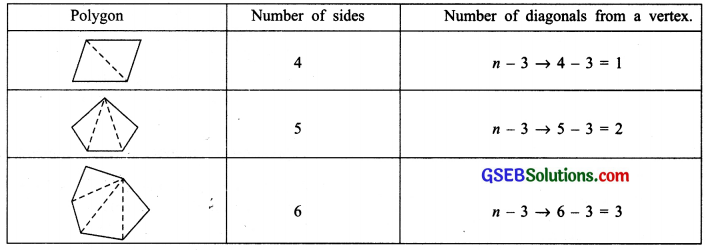# GSEB Solutions Class 7 Maths Chapter 12 Algebraic Expressions Ex 12.4

Gujarat Board GSEB Textbook Solutions Class 7 Maths Chapter 12 Algebraic Expressions Ex 12.4 Textbook Questions and Answers.

## Gujarat Board Textbook Solutions Class 7 Maths Chapter 12 Algebraic Expressions Ex 12.4Question 1.
Observe the patterns of digits made from line segments of equal length. You will find such segmented digits on the display of electronic watches or calculators.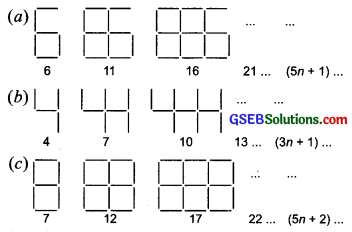If the number of digits formed is taken to be n, the number of segments required to form n digits is given by the algebraic expression appearing on the right of each pattern. How many segments are required to form 5, 10, 100 digits of the kind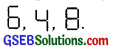Solution:
(a)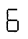∵ Expression for number of line segments to form ‘n’ figures of the kindis 5n + 1.
∴ For 5 figures, line segments = 5 x 5 + 1 = 25 + 1= 26
For 10 figures, line segments = 5 x 10 + 1 = 50 + 1 = 51
For 100 figures, line segments = 5 x 100 + 1 = 500 + 1 = 501

(b)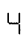∵ Expression for number of line segments to form ‘n’ figures of the kindis 3n + 1.
∴ For 5 figures, line segments = 3 x 5 + 1 = 15 + 1 = 16
For 10 figures, line segments = 3 x 10 + 1 = 30 + 1 = 31
For 100 figures, line segments = 3 x 100 + 1 = 300 + 1 = 301

(c)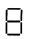∵ Expression for number of line segments to form ‘n’ figures of the kindis 5n + 2
∴ For 5 figures, line segments = 5 x 5 + 2 = 25 + 2 = 27
For 10 figures, line segments = 5 x 10 + 2 = 50 + 2 = 52
For 100 figures, line segments = 5 x 100 + 2 = 500 + 2 = 502Question 2.
Use the given algebraic expression to complete the table of number patterns.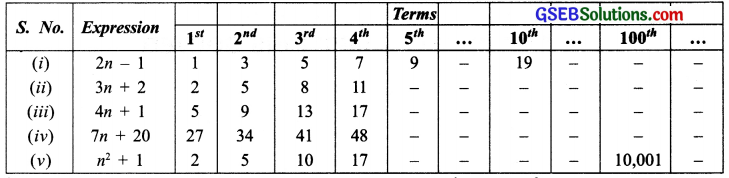Solution:
(i) For the expression 2n – 1
The 100th term = 2 x 100 – 1
= 200 – 1 = 199

(ii) For the expression 3n + 2,
The 5th term = 3 x 5 + 2 = 15 + 2= 17
The 10th term = 3 x 10 + 2 = 30 + 2 = 32
The 100th term = 3 x 100 + 2 = 300 + 2 = 302

(iii) For the expression An + 1,
The 5th term = 4 x 5 + 1 = 20 + 1 = 21
The 10th term = 4 x 10 + 1 = 40 + 1 = 41
The 100th term = 4 x 100 + 1 = 400 + 1 = 401

(iv) For the expression In + 20,
The 5th term = 7 x 5 + 20 = 35 + 20 = 55
The 10th term = 7 x 10 + 20 = 70 + 20 = 90
For 100th term = 7 x 100 + 20 = 700 + 20 = 720

(v) For the expression n² + 1,
The 5th term = 5² + 1 = 25 + 1 = 26
The 10th term = 10² + 1 = 100 + 1 = 101

Note:
1. We can have some patterns with the help of dots also, such as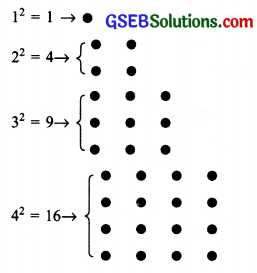2. We can have some geometrical patterns as: